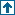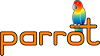parrotcode: pir.y Contents | Compilers

pir.y

# DESCRIPTIONThis is a complete rewrite of the parser for the PIR language.

TODO: 1. [done 9/8/8] fix argument stuff related to the :named flag. 2. [done 9/8/8] fix parameter stuff 3. [done 17/8/8] clean up back-end a bit (refactoring, consting) 4. improve memory management (free it!) 5. test 6. [done 12/8/8] write vanilla register allocator 7. generate PBC, using Parrot_PackFile (and related) data structures. This needs linkage to libparrot, which seems to fail.

# Constant folding routines.`static constant *fold_i_i(yyscan_t yyscanner, int a, pir_math_operator op, int b)`

Evaluates the expression `a op b` and returns a constant node containing the result value. Both `a` and `b` are integer values.

`static constant *fold_n_i(yyscan_t yyscanner, double a, pir_math_operator op, int b)`

Same as `fold_i_i`, except `a` is of type double.

`static constant *fold_i_n(yyscan_t yyscanner, int a, pir_math_operator op, double b)`

Same as `fold_i_i`, except `b` is of type double.

`static constant *fold_n_n(yyscan_t yyscanner, double a, pir_math_operator op, double b)`

Same as `fold_i_i`, except that both `a` and `b` are of type double.

`static constant *fold_s_s(yyscan_t yyscanner, char *a, pir_math_operator op, char *b)`

Evaluate the expression `a op b`, where both `a` and `b` are strings. Only the concatenation and comparison operators are implemented; other operators will result in an error.

`static int evaluate_n_n(double a, pir_rel_operator op, double b)`

Compare `a` with `b` according to the relational operator `op`. `op` can be `<!=`>, `<==`>, `<`, `<=`, `>` or `>=`.

`static int evaluate_s(char *s)`

Evaluate a string in boolean context; if the string's length is 0, it's false. If the string equals "0", ".0", "0." or "0.0", it's false. Otherwise, it's true.

`static char *concat_strings(char *a, char *b)`

Concatenates two strings into a new buffer; frees all memory of the old strings. The new string is returned.